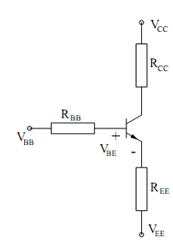## Analysis of the BJT DC Bias Conditions Assignment Help

Assignment Help: >> Transistor Amplifier Circuits - Analysis of the BJT DC Bias Conditions

Analysis of the BJT DC Bias Conditions:

A typical DC bias circuit for biasing the transistor to behaves as an amplifier may be usually simplified by invoking Thevenin's theorem. Therefore, looking out of the 3 terminals of the BJT, the Thevenin's equivalent as illustrated in the circuit in Figure is easily conceivable.Figure: Basic Transistor Bias Circuit

To analyze such an equivalent circuit, we write a loop equation for the base-emitter loop.

VBB  - VEE  = I B RBB  +VBE  + I E REE

Then by using the relation IC = β IB =α IE, we may express IB and IE as functions of the

current IC , as follows :

V BB       - V EE  = IC  / β  R BB      +V BE     + (IC  / α  )REE

We can then solve for IC.

IC  = VBB  - VEE  - VBE / RBB (IC    / β) + ( IC/  α ) REE

An "educated guess" of the value of VBE should be made to evaluate this. Typical values are 0.7V for IC transistors, 0.6-0.65 V for low-power discrete transistors, and 0.5-0.6 V for higher-power discrete transistors.

At last, we may check whether or not the transistor is in active mode. For the active mode, we must check that VCB > 0, which may be done as follows :

VCB  = VC  - VB  = (VCC  - IC RCC ) - (VBB  - I B RBB )

or         = (VCC  - IC RCC ) - (VEE  + I E REE  + VBE )